Lecture notes for ZOO 4400/5400 Population Ecology

Lecture 32 (19-Apr-13)

The spread of infectious diseases (continued).

Last time, I introduced a simple difference (discrete) equation model for the spread of an infectious disease.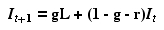Eqn 32.1 (= Eqn 31.2)
which has the following equilibrium value (I*)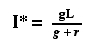Eqn 32.2 (= Eqn 31.8)

What does equilibrium mean here?  It means that there will be no change in the infection level -- that is the rate of addition of new infections will be balanced by the recovery rate (a population growth analog would be dN/dt = 0 when births = deaths) .

We also solved for some aspects of the graph of It as a function of time.  We know its Y-intercept, given by: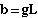{Y-intercept}                    Eqn 32.3 (= Eqn 31.4)
and its slope given by
m = (1 - g - r)        {slope}                           Eqn 32.4 (= Eqn 31.5)
Working with empirical data for the observed spread of an infectious disease.

Let's say we have an empirically observed plot of It values over time. We have only two equations (Eqn 32.3 = Eqn 31.4 and Eqn 32.4 = 31.5) for three unknowns, L, g, and r -- too few equations to solve for the unknowns. We can, however, use some tricks, based on other knowledge about constraints on the system, to obtain estimates of these unknown parameters from the data.

First, let's find the equilibrium solution from the observed data. Remember from Eqns 32.3 and 32.4 that gL and (1 - g - r) are the intercept and slope respectively. But the equilibrium [Eqn 32.2] is just the intercept (gL) divided by 1 minus the slope [1 - (1 - g - r) = g + r], so we can calculate the equilibrium value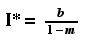Eqn 32.5
If we calculate the slope from ordinary least squares regression (that is, by analyzing the numerical values of the data points statistically) or simply by using a cruder rise-over-run approach on a graph, we'll then be able to calculate the equilibrium level of infection. Next let's look at reasonable bounds on g.
From Eqn 32.3Eqn 32.6 (= Eqn 32.3 = Eqn 31.4)
so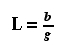Eqn 32.7
But we know that 0 and 1.0 are the bounds on the possible values of L, so we can rewrite Eqn  32.7 as an inequality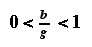Eqn 32.8
Look just at the b/g < 1 and multiply both sides by g to get b < g which we can reverse as:
g > b                                             Eqn 32.9

That is, g (the infection rate per unit time) will be greater than the Y-intercept of our empirical plot.  We now have a lower bound for g. Let's use similar logic to calculate an upper bound. Remember from Eqn 32.4 that the slope is given by

m = (1-g-r)      {slope}                      Eqn 32.10 (= Eqn 32.4 = Eqn 31.5)
which can be rearranged to solve for r as
r = 1-g-m                                            Eqn 32.11
As for L, r lies in the interval 0 to 1.0, so
0 < 1-g-m < 1                                    Eqn 32.12
Now just move the g to the left of the inequality (and ignore the fact that it is, of course < 1), so that we now know that
g < 1-m                                               Eqn 32.13

That is, we now know that the upper limit for g is given by 1 minus the slope (m) of our empirical plot.

Combine Eqns 32.9 and 32.13 together for the upper and lower bounds on g

b < g < 1-m                                         Eqn 32.14
Let's make the reasonable assumption that the possible estimates of g are normally distributed (i.e., the values fit a bell-shaped curve) over the interval from b to 1 - m. The maximum likelihood estimator of g is then the midpoint of that interval. [§§ We could use a more complex maximum likelihood estimator (MLE) for other kinds of distributions §§]. This gives us an estimate of g as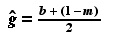Eqn 32.15
where the "hat" over the variable denotes the fact that it is an estimate.  That "solved" for one of the three (too many) unknowns.  Now we can solve for the other two (just enough) unknowns. Substituting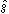into Eqn 32.11 we get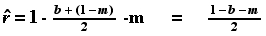Eqn 32.16
and substitutinginto Eqn 32.7 we get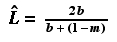Eqn 32.17
Let's say that our observed data had an intercept, b, of 0.1 and slope, m, of 0.4. We then obtain estimates of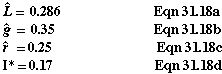[Note: all the above should be Eqn 32.18 not 31.18] We have used knowledge of constraints on the system to calculate equilibrium values and parameter estimates from relatively little information. From the observed infection data we have been able to estimate

1) the rate of spread (g),
2) the rate of recovery (r), and
3) the upper level of proportion infectable (L).

Having an estimate of any one of those three previously unknown parameters could be very useful in managing a population subject to infectious disease.  Estimating them directly would be very difficult. Doing the empirical plot of It+1 against It is much more feasible, and is all we really need.

References:
Huckfeldt, R.R., C.W. Kohfeld, and T.W. Likens. 1993. Dynamic Modeling: An Introduction.
Quant. Applic. Soc. Sciences No. 27.
Sage University Press, Thousand Oaks, CA

§§§§ §§§§§§§§§§§ §§§§§§§§§§§§§ §§§§§§§§§§§§§§§§§§§§§§§§§§§ §§§§§§§§